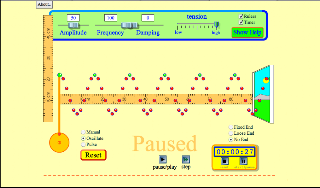## Thursday, June 30, 2011

### Worksheet 1 General Properties of Waves [Example 3] -Vivek Kun Yao

What affects the speed of the Wave?

With the following pictures, we can prove if speed changes if we either change the frequency or the amplitude. Here is a picture of our control.

Control:Above is a picture of the control when both the Amplitude and the
Frequency is at 50.

Frequency Increased:Above is a picture of what happens if we changed the Frequency to 100. W
e have also calculated the time of a full cycle.

Amplitude Increased:Above is a picture of what happens when we increase the Amplitude to 1
00 and decrease the Frequency back to 50. We also calculated the timing of a full cycle.

Tension Changed:Finally, this is a screenshot of what happens when we changed the tension. The time taken for a complete wave is 55 seconds.

Over here we shall calculate the speed of the wave when either the amplitude or the frequency has increase.

Speed of Control.
Speed = Distance/Time
= 90/55
= 1.636
= 1.64 cm/s (3 s.f.)

Speed when Amplitude is changed.
Speed = Distance/Time
= 90/55
= 1.636
= 1.64 cm/s (3 s.f.)

Speed when Frequency is changed.
Speed = Distance/Time
= 90/27
= 3.333
= 3.33 cm/s (3 s.f.)

Speed when Tension changed.
Speed = Distance/Time
= 90/55
= 1.636
= 1.64 cm/s (3 s.f.)

Control:

90cm/timetaken

Higher Frequency:

90cm/timetaken

When the frequency is higher than 50 the speed changes as the wavelength of the wave changes. This affects the speed too. This shows that when there is a higher frequency the speed of the wave changes.

Control:

90cm/timetaken

Higher Amplitude:

90cm/timetaken

When the amplitude changes it changes in the why direction so the time taken and the wavelength or period do not get affected, thus the speed of the wave is still the same.

Control:

90cm/timetaken

Lower Tension:

90cm/timetaken

When the tension is lower the wave moves through the string in the same speed as when the tension is higher. Thus, the elasticity does not help the wave move easier. This results in the same speed when the wave is on a less elastic speed compared to a more elastic speed.

Vivek
Kun Yao
S2-05

#### 1 comment:

1.Is the same distance used for the wave to travel when doing the part on frequency?